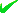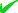News By Tag
Industry News
News By Place

Country(s)

Industry News

May 2023
SuSaFrThWeTuMo
28272625242322

# 1/f Noise in Nanowires by Quantum Mechanics

Quantum mechanics that requires the conservation of Joule heat by the creation of charge instead of an increase in temperature produces 1/f noise by a train of step changes in power similar to a Markov chain in a stochastic stationary process
By: Thomas Prevenslik

1/f Noise Spectra in Nanowires
Feb. 6, 2012 - PRLog -- Background

The 1/f noise commonly observed in the measurements of power in electronic circuits comprising semiconductors, resistors, vacuum tubes, and transistors. Other processes in the natural world also show this behavior including stock prices and music suggest  a generic origin might exist, but no generally recognized physical explanation of 1/f noise has been proposed. Indeed, the ubiquity of 1/f noise is one of the oldest puzzles of contemporary physics.

Of interest is the origin of the 1/f noise in nanoelectronics, say in nanowires of tin oxide  having submicron diameters of 10-50 nm and lengths from 1-10 microns. Historically, electronic noise or white noise from vacuum tubes was discovered  by Johnson and Nyquist in 1925. White noise has a constant power spectrum generated by the thermal agitation of charges inside a resistor regardless of any applied voltage. In contrast, 1/f noise sometimes called pink noise differs in that under applied voltage the frequency f of the power spectrum is not constant, but rather increases as f is lowered, i.e., having a slope of -1 on a log-log plot or with the frequency f raised to the -1 exponent.

Over the past 80 years, explanations of 1/f noise have proved  elusive. Schottky proposed a mathematical model that simulated an exponentially decaying current pulse I caused by the release of electrons from the cathode of a vacuum tube, i.e., I = Io×exp-bt, where Io is the initial step, b is the relaxation rate and t is time. For a train of such pulses at an average rate, the power spectrum is of the Lorentzian form which is constant near f = 0 and for large f is proportional to 1/f2 with a narrow transition region where the power spectrum resembles that of 1/f noise. Later, Bernamont pointed out only a superposition of such processes with a variety of relaxation rates would yield 1/f noise for a reasonable range of frequencies. Given that 1/f noise as a stationary process has a mean and variance that do not change over time, Erland et al.  showed observations of such processes may be modeled as a Markov chain in which one observation depends stochastically only on the immediately previous one.

But what is the physical process in the Markov chain that underlies 1/f noise in nanowires?

Nanoelectronics in general and nanowires in particular are nanostructures that by QM create charge  upon dissipating Joule heat. QM stands for quantum mechanics and Joule heat is given by I2R, where R is resistance. QM precludes atoms in nanostructures from having the heat capacity necessary to conserve Joule heat by an increase in temperature, and therefore the Joule heat under EM confinement is induced by QED to produce non-thermal radiation that by Einstein’s photoelectric effect creates charge inside the nanostructure. EM stands for electromagnetic and QED for quantum electrodynamics. In this regard, QED induced charge in nanowires finds similarity with memristors  including the Ovshinsky Effect in PCRAM devices whereby charge is created in amorphous GST films from excitons (holes and electrons) by the photoelectric effect that locally reduce the film resistance to allow encoding of information. See http://www.prlog.org/11649780-the-ovshinsky-effect-by-qua...

Physical Markov Process

In nanowires, QED induces the Joule heat to produce a train of step changes in QED power each of which decay in the otherwise macroscopic circuit. In this way, a Markov process is formed with each step change in power effectively depending on the previous one. However, stochastic Markov processes may not be necessary. Indeed, the 1/f spectrum may be considered deterministic and caused by a steady step change in power produced by simply passing current through the nanowire.     .

Fourier Transforms

The Fourier transform (FT) of power as an arbitrary step function ta in time gives f -(a+1) in the frequency domain, where a is a rational number. See "An Interesting Fourier Transform - 1/f Noise," at http://www.dsprelated.com/showarticle/40.php For a = 0, the FT of a step is 1/f and as depicted in relation to the reference 1/f and 1/f2 spectra in the thumbnail. Clearly, the FTs of ta for a = +0.5 and -0.25 are not sufficient to tightly bracket the 1/f noise, and therefore numerical FTs of ta for a = -1.1 and – 0.9 are required.

Summary

Upon absorption of Joule heat in the nanowire, QED induces the creation of photons under the EM confinement across the nanowire diameter d. The QED photons have Planck energy E = hc/2nd, where h is Planck’s constant, c is the speed of light, and n is the refractive index of the nanowire. Typically, E > 6 eV, and therefore charge is created from excitons (holes and electrons) by the photoelectric effect. Although the exciton charges balance each other, the nanowire conductance increases. Under the  applied voltage, the current increases to produce the step change in QED induced power.

The step change in QED power occurs abruptly. For a nanowire of length L, the QED induced charge velocity is v = mu×V/L, where mu is the mobility of the excitons and V the voltage across the wire. Charge velocities are high in nanowires. For V = 1 volt, L = 1 micron, and mu = 0.02 m2/Volt-s, velocities of 20,000 m/s correspond to a rise time L/v = 50 ps. Thereafter, the QED power is dissipated in the circuit, and therefore the 1/f spectrum is produced by a continuous train of steps in QED power. See  “1/f Noise in Nanowires" at http://www.nanoqed.org , 2012.

Conclusions

1. Charge continuously created in nanowires by QM is consistent with a Markov chain of step changes in power in the manner of a stochastic stationary process. However, a Markov chain may not be necessary as a step change in power occurs naturally upon passing current through nanowires.

2. The physical process that underlies the 1/f spectrum in nanowires begins with the QED induced conservation of Joule heat by non-thermal EM radiation instead of by an increase in temperature. The EM radiation having the Planck energy necessary to create excitons by the photoelectric effect then lowers the nanowire resistance that momentarily increases the current to produce a step change in QED power. The 1/f noise is produced as the step changes in QED power are continuously repeated as current flows through the nanowire.

References

 S. Erland, P. E. Greenwood, L. M. Ward, “1/f noise” is equivalent to an eigenstructure power relation,” EPL, 95 (2011) 60006.
 S. Ju, et al., ”1/f noise of SnO2 nanowire transistors,” Appl. Phys. Lett., Vol 92 (2008) 243120.
 H. Nyquist, “Thermal agitation of electric charge in conductors*, Phys. Rev., Vol. 32 (1928) 110-113.
 T. Prevenslik, “Quantum Mechanics and Nanoelectronics,” ICMON 2011 : International Conference on Microelectronics, Optoelectronics, and Nanoelectronics, Venice, November 28-30, 2011

# # #

Classically, thermal EM radiation conserves heat by an increase in temperature. But in nanostructures, temperature increases are forbidden by quantum mechanics. QED radiation explains how heat is conserved by the emission of nonthermal EM radiation that charges the nanostructure by the photoelectric effect.
End
Source Email : Thomas Prevenslik : ***@gmail.com: 15697 : 1/f Noise, Nanoelectronics, Quantum Mechanics, Fourier transforms, Markov process, Lorentzains, Quantum Electrodynamics : Electronics, Semiconductors, Research : Youngwood - Pennsylvania - United States : Reports
Account Email AddressAccount Phone NumberDisclaimer     Report Abuse